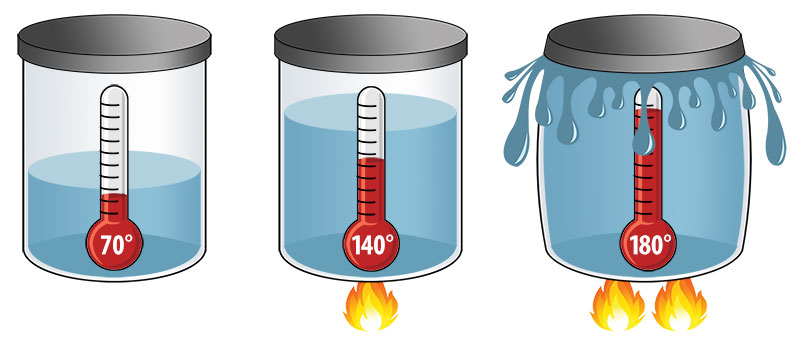# Volume and Heat

Time for some practical applications of all this solid, liquid, gas stuff. We can manipulate the properties of many materials by varying the amount of energy the particles have.The more you heat something, the greater the pressure outwards becomes as the volume grows.

When we did the demo lesson on air, you already observed this with the can crush and balloon inversion. Copy down the following section

Volume is dependent on heat/energy.
IF the temperature is increased, then the pressure increases
IF the temperature is decreased, then the pressure decreases

When heat is applied to a solid they expand to occupy more space.
When heat is removed from a solid they shrink to occupy less space.

## Thermal Expansion and Burners

Aim: To see how heating a metal ring/ball effects it’s size
Hypothesis: If I heat the ball then ……(your assumption here)…….
Independent Variable: I am changing _______________________
Dependent Variable: I am measuring _______________________
Control Variable: I am keeping ____________________________ the same

Method: 1. Get the ball and rod setup with a Bunsen burner and tongs.
2. Test to see if the ball fits through the ring
3a. If the ball does fit, heat ball up in the burner
3b. If the ball doesn’t fit, heat ring up in the burner
4. Use tongs and carefully see if the 2 still fit or not

Discussion
What did you observe?

Could you see the size difference in the ball or ring once heated?

How would you describe what happened to a friend?

How would you describe what happened using particle theory?